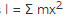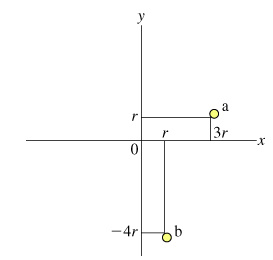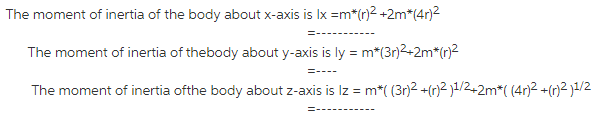# On which of the following does the moment of inertia of an object depend?

1.On which of the following does the moment of inertia of an object depend?
linear speed
linearacceleration
angularspeed
angularacceleration
totalmass
shape anddensity of the object
location of the axis of rotation

2.Consider the system of two particles, a and b, shown in the figure.Particle a has mass m, and particle b has mass 2m.

Find the moment of inertia Ix of particle a with respect to the x axis (that is, if the x axis is the axis of rotation), the moment of inertia I_y of particle a with respect to they axis, and the moment of inertia Iz of particle a with respect to the z axis (the axis that passes through the origin perpendicular to both the x and y axes).

The moment ofinertia of the system is